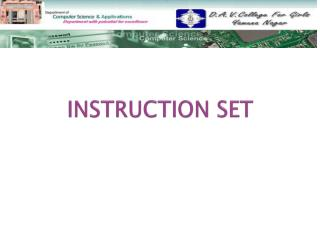DownloadDownload PresentationINSTRUCTION SET

# INSTRUCTION SET

Télécharger la présentation## INSTRUCTION SET

- - - - - - - - - - - - - - - - - - - - - - - - - - - E N D - - - - - - - - - - - - - - - - - - - - - - - - - - -
##### Presentation Transcript

1. INSTRUCTION SET

2. TOPICS TO BE DISCUSSED INSTRUCTION SET VARIOUS IMPORTANT REGISTERS ADDRESSING SCHEMES EXAMPLE

3. INSTRUCTION SET A instruction set is a collection of all the instructions a CPU can execute. ELEMENTS OF AN INSTRUCTION Opcode Address of an operand Reference for storing the result Reference for the next instruction

4. VARIOUS IMPORTANT REGISTERS • Accumulator register(AC) • Memory Buffer Register(MBR) • Program Counter(PC) • Instruction Register(IR) • Memory Address Register(MAR) • General Purpose Register • Condition Codes Registers • Status and Control Registers

5. ADDRESSING SCHEMES There are various addressing schemes depending upon the architecture of computer. • 4-address scheme • 3-address scheme • 2-address scheme • 1-address scheme • 0-address(or no-address) scheme

6. 4-address scheme This instruction consists of following parts: • Operation code • Two address for two operands • Address of memory location where result of operation is to be stored • Address of memory location containing the next instruction Op-code Address-1 Address-2 Address of result Address of next instruction

7. 3-address scheme This instruction consists of following parts: • Operation code • Two address for two operands • Address of memory location where result of operation is to be stored Op-code Address-1 Address-2 Address of result

8. 2-address scheme This instruction consists of following parts: • Operation code • Two address for two operands. The result is placed in one of the specified address. Op-code Address-1 Address-2

9. 1-address scheme In this instruction only one operand is specified in the instruction, the other operand is stored in accumulator. The results are stored in accumulator. Op-code Address-1

10. 0-address scheme This instruction do not contain any operand address. The operand addresses are implied. They are also called stack instructions. Op-code

11. EXAMPLE…… F=(R+S)*(U+V) Solution….. Three address instructions: ADD R1,R,S R1<-M[R]+M[S] ADD R2,U,V R2<-M[U]+M[V] MUL F,R1,R2 M[F]<-R1+R2

12. Two Address instructions MOV R1,R R1<-M[R] ADD R1,S R1<-R1+M[S] MOV R2,U R2<-M[U] ADD R2,V R2<-R2+M[V] MUL R1,R2 R1<-R1*R2 MOV F,R1 M[F]<-R1

13. One-Address Instructions LOAD R AC<-M[R] ADD S AC<-AC+M[S] STORE T M[T]<-AC LOAD U AC<-M[U] ADD V AC<-AC+M[V] MUL T AC<-AC*M[T] STORE F M[F]<-AC (T=Temporary Location)

14. Zero Address Instructions PUSH R TOS<-R PUSH S TOS<-S ADD TOS<-(R+S) PUSH U TOS<-U PUSH V TOS<-V ADD TOS<-(U+V) MUL TOS<-(R+S)*(U+V) POP F M[F]<-TOS (TOSTop of Stack)

15. THANKS…….